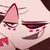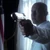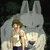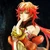24,830 Pages

## Summary

The ability to manipulate mathematics.

## Types

• Abstract manipulation of mathematics: The ability to manipulate mathematics in its abstract form. This means that things like the way reasoning is performed, the mathematical notation, inference rules and which assumptions and models are used when doing math can be changed, but the phenomenons of reality described through the math are unaffected.
• Applied manipulation of mathematics: The ability to manipulate real phenomenons by changing the mathematical rules through which they are described.

## Possible Uses

• Physics Manipulation: Most laws of physics are described by mathematical equations. Through the change of what those equations mean or change of the equations themselves the described phenomena would have to change accordingly, assuming that the description of them stays true even if changed or in other words if the equations aren't just changed on paper, but in reality.
• Quantity Manipulation: The study of how much there is of something usually is viewed as subject of mathematics. By manipulating the subject itself the results of measuring the quantity of something can be changed, effectively making it so that there is more of something or less.
• Operation Manipulation: By changing the way mathematical operations work real phenomena described through them may have different results. For example by changing the laws so that 1+1=3, it could be made so that if one apple is taken and another one put to it, the resulting amount of apples is three apples. Another application of this is to forcefully apply mathematical operations to reality, for example building the sum of two things making them fuse together.
• Dimensional Manipulation: Since dimensions are a mathematical concept, a user of this ability might be capable of changing the attributes of dimensions and the concept of them in itself. One possible application would for example changing 5 dimensional things to 3 dimensional and vice versa.
• Logic Manipulation: Since mathematics builds upon, includes and extends logic, changing mathematics can have an effect on the underlying logical concepts. For example by creating a mathematical contradiction and through the principle of "ex falso sequitur quodlibet" the validity and usefulness of logic could be undermined.
• Spatial Manipulation: Since concepts like distance and properties of space are in abstract form part of the mathematical studies changing the mathematics describing those could also have effect on the real properties of space.
• Manipulation of form: The user might be capable of changing the form, volume, area and similar of things, as those are also mathematical properties of objects.
• Probability Manipulation: As the probability for something to happen is usually expressed as mathematical value, a user might be capable of influencing probability by changing that value.

## Limitations

• Users might only have certain parts of those abilities.

## Users

• SCP-033
• Othinus and other magic gods

## Start a Discussion Discussions about Mathematics Manipulation

•#### The biggest number Part 1

40 messages
•2!
•My love for you is Grahams! so it can't named by any human langueges LoL LMSFAO.
•#### Fallen London Stuff

96 messages
•update this https://vsbattles.wikia.com/wiki/Judgements
•They need the addition that their light kills Souless beings and the undead, and also that they control the chain of life
Community content is available under CC-BY-SA unless otherwise noted.